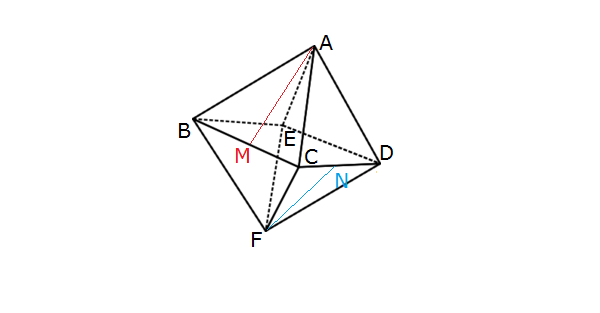Geometry

# 3D Coordinate Geometry - Skew Lines

Calculate the distance between the following two lines: $r = \frac{x-1}{3}=\frac{y-4}{-1}=\frac{z+1}{4}$ and $\begin{cases} x = 4 +\lambda \\ y = 1 \\ z= 8 + 2\lambda \end{cases} .$In the above regular octahedron $ABCDEF,$ $M$ and $N$ are the midpoints of $\overline{BC}$ and $\overline{CD},$ respectively. If $\theta$ is the angle between $\overrightarrow{NF} \text{ and } \overrightarrow{MA},$ what is the value of $\cos \theta?$Count the number of pairs of edges in the above cuboid that are skew.

If the following two lines are not skew, what is the value of the non-zero variable $u:$ $\begin{cases} l_1: \frac{x}{2}=\frac{y}{3}=\frac{z-2}{2} \\\\ l_2 :\frac{x}{2}=\frac{y}{4}=\frac{z-u}{u}? \end{cases}$

Find the distance between the following two lines:

$\begin{cases} l_1: x=-y=8-z \\\\ l_2: x=y \text{ and } z=-10. \end{cases}$

×